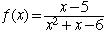I have the problem- f(x)= X-5/X2+X-6 and I have to find the vertical asymtope, horizontal asymtope, x-intercept, and y-intercept. And graph the problem. I am having problems finding the y-intercept. I am a student asking a question from my book. Hi, The y-intercepts are the points where the graph crosses the y-axis. Points on the y-axis are points of the form (0,y), that is the x-coordinate is zero. In the expressionIf you set x = 0 you get f(x) = 5/6. Thus the only y-intercept is (0, 5/6). The x-intercepts arre the points where the graph crossed the x-axis. These are points of the form (x,0), that is the y-coordinate must be zero. Your expression  x-5/x2+x-6 can only have a value of zero when the numerator is zero and the denominator is not zero. This happens when x = 5 and hence the only x-intercept is (0,5) Cheers, Harley Go to Math Central